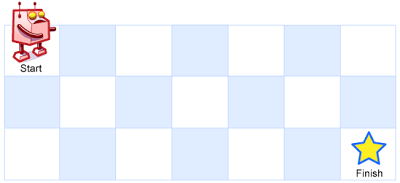## Description

Unique Paths

A robot is located at the top-left corner of a m x n grid (marked ‘Start’ in the diagram below).

The robot can only move either down or right at any point in time. The robot is trying to reach the bottom-right corner of the grid (marked ‘Finish’ in the diagram below).

How many possible unique paths are there?Above is a 7 x 3 grid. How many possible unique paths are there?

Note: m and n will be at most 100.

Example 1:

``````Input: m = 3, n = 2
Output: 3
Explanation:
From the top-left corner, there are a total of 3 ways to reach the bottom-right corner:
1. Right -> Right -> Down
2. Right -> Down -> Right
3. Down -> Right -> Right
``````

Example 2:

``````Input: m = 7, n = 3
Output: 28
``````

`m x n`的方格中，从左上角走到右下角的不同路径有几种？（只允许向右和向下走）

## Solution

`m x n`的方格要从左上角走到右下角，需要经过`m - 1``Right``n - 1``Down`操作。 所以总共是`m + n - 2`次操作，而操作顺序的不同，则对应路径不同。

 `````` 1 2 3 4 5 6 7 8 9 10 11 12 13 14 15 16 17 18 19 20 21 22 23 `````` ``````func uniquePaths(m int, n int) int { total := (m + n - 2) option := n - 1 // C(3, 1) 与 C(3, 2)相等 if m-1 < option { option = m - 1 } if option <= 0 { return 1 } // 计算C(m + n - 2, option) pp, qq := 1, 1 for i := 1; i <= option; i++ { pp *= total qq *= i total-- } return pp / qq }``````